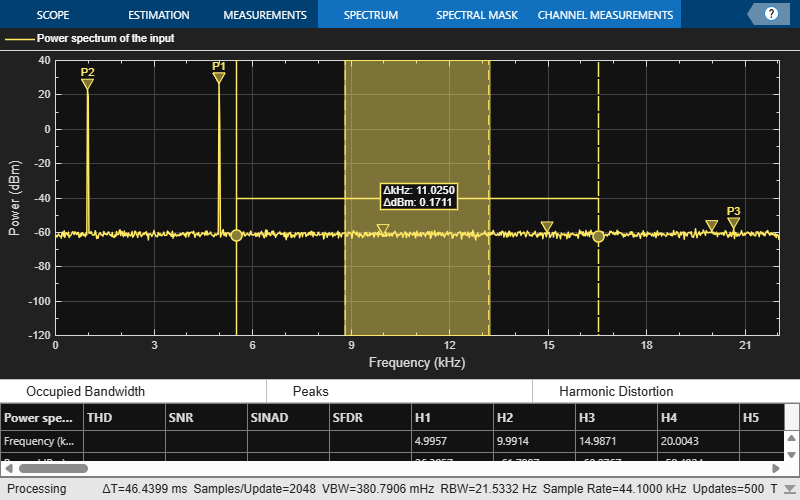# getMeasurementsData

Get the current measurement data displayed on the spectrum analyzer

## Syntax

``data = getMeasurementsData(scope)``
``data = getMeasurementsData(scope,'all')``

## Description

example

````data = getMeasurementsData(scope)` returns a data table about the current spectrum analyzer measurements in use.```
````data = getMeasurementsData(scope,'all')` returns a data table about all spectrum analyzer measurements for the current time step.```

## Examples

collapse all

Compute and display the power spectrum of a noisy sinusoidal input signal using the `dsp.SpectrumAnalyzer` System object. Measure the peaks, cursor placements, adjacent channel power ratio, distortion, and CCDF values in the spectrum by enabling the following properties:

• `PeakFinder`

• `CursorMeasurements`

• `ChannelMeasurements`

• `DistortionMeasurements`

• `CCDFMeasurements`

Initialization

The input sine wave has two frequencies: 1000 Hz and 5000 Hz. Create two `dsp.SineWave` System objects to generate these two frequencies. Create a `dsp.SpectrumAnalyzer` System object to compute and display the power spectrum.

```Fs = 44100; Sineobject1 = dsp.SineWave('SamplesPerFrame',1024,'PhaseOffset',10,... 'SampleRate',Fs,'Frequency',1000); Sineobject2 = dsp.SineWave('SamplesPerFrame',1024,... 'SampleRate',Fs,'Frequency',5000); SA = dsp.SpectrumAnalyzer('SampleRate',Fs,'Method','Filter bank',... 'SpectrumType','Power','PlotAsTwoSidedSpectrum',false,... 'ChannelNames',{'Power spectrum of the input'},'YLimits',[-120 40],'ShowLegend',true);```

Enable Measurements Data

To obtain the measurements, set the `Enable` property of the measurements to `true`.

```SA.CursorMeasurements.Enable = true; SA.ChannelMeasurements.Enable = true; SA.PeakFinder.Enable = true; SA.DistortionMeasurements.Enable = true;```

Use `getMeasurementsData`

Stream in the noisy sine wave input signal and estimate the power spectrum of the signal using the spectrum analyzer. Measure the characteristics of the spectrum. Use the `getMeasurementsData` function to obtain these measurements programmatically. The `isNewDataReady` function indicates when there is new spectrum data. The measured data is stored in the variable `data`.

```data = []; for Iter = 1:1000 Sinewave1 = Sineobject1(); Sinewave2 = Sineobject2(); Input = Sinewave1 + Sinewave2; NoisyInput = Input + 0.001*randn(1024,1); SA(NoisyInput); if SA.isNewDataReady data = [data;getMeasurementsData(SA)]; end end```The right side of the spectrum analyzer shows the enabled measurement panes. The values shown in these panes match with the values shown in the last time step of the `data` variable. You can access the individual fields of `data` to obtain the various measurements programmatically.

Compare Peak Values

Peak values are obtained by the `PeakFinder` property. Verify that the peak values obtained in the last time step of `data` match the values shown on the spectrum analyzer plot.

`peakvalues = data.PeakFinder(end).Value `
```peakvalues = 3×1 26.9850 24.1735 -52.3506 ```
`frequencieskHz = data.PeakFinder(end).Frequency/1000`
```frequencieskHz = 3×1 4.9957 0.9905 7.8166 ```

Compute and display the power spectrum of a noisy sinusoidal input signal using the Spectrum Analyzer block. Measure the peaks, cursor placements, adjacent channel power ratio, distortion, and CCDF values in the spectrum by enabling these block configuration properties:

• PeakFinder

• CursorMeasurements

• ChannelMeasurements

• DistortionMeasurements

• CCDFMeasurements

Open and Inspect the Model

Filter a streaming noisy sinusoidal input signal using a Lowpass Filter block. The input signal consists of two sinusoidal tones: 1 kHz and 15 kHz. The noise is white Gaussian noise with zero mean and a variance of 0.05. The sampling frequency is 44.1 kHz. Open the model and inspect the various block settings.

```model = 'spectrumanalyzer_measurements.slx'; open_system(model) ```Access the configuration properties of the Spectrum Analyzer block using the `get_param` function.

```sablock = 'spectrumanalyzer_measurements/Spectrum Analyzer'; cfg = get_param(sablock,'ScopeConfiguration'); ```

Enable Measurements Data

To obtain the measurements, set the `Enable` property of the measurements to `true`.

```cfg.CursorMeasurements.Enable = true; cfg.ChannelMeasurements.Enable = true; cfg.PeakFinder.Enable = true; cfg.DistortionMeasurements.Enable = true; ```

Simulate the Model

Run the model. The Spectrum Analyzer block compares the original spectrum with the filtered spectrum.

```sim(model) ```The right side of the spectrum analyzer shows the enabled measurement panes.

Using `getMeasurementsData`

Use the `getMeasurementsData` function to obtain these measurements programmatically.

```data = getMeasurementsData(cfg) ```
```data = 1x5 table SimulationTime PeakFinder CursorMeasurements ChannelMeasurements DistortionMeasurements ______________ ____________ __________________ ___________________ ______________________ {[0.9985]} [1x1 struct] [1x1 struct] [1x1 struct] [1x1 struct] ```

The values shown in measurement panes match the values shown in `data`. You can access the individual fields of `data` to obtain the various measurements programmatically.

Compare Peak Values

As an example, compare the peak values. Verify that the peak values obtained by `data.PeakFinder` match with the values seen in the `Spectrum Analyzer` window.

```peakvalues = data.PeakFinder.Value frequencieskHz = data.PeakFinder.Frequency/1000 ```
```peakvalues = 26.9663 26.3212 -3.5677 frequencieskHz = 15.0015 1.0049 12.3457 ```

Save and Close the Model

```save_system(model); close_system(model); ```

## Input Arguments

collapse all

Spectrum analyzer you want to query. Specify a `dsp.SpectrumAnalyzer` System object or a `SpectrumAnalyzerConfiguration` object for a spectrum analyzer block.

## Output Arguments

collapse all

When you specify `'all'`, a measurements table containing the following fields is returned:

FieldDescription
`SimulationTime`Simulation time
`PeakFinder`Peak finder data
`CursorMeasurements`Cursor measurements data
`ChannelMeasurements`Channel measurements data
`DistortionMeasurements`Distortion measurements data
`CCDFMeasurements`CCDF measurements data

When you do not specify `'all'`, the data table contains only the spectrum analyzer measurements currently in use.

### Blocks

Introduced in R2018b

Watch now